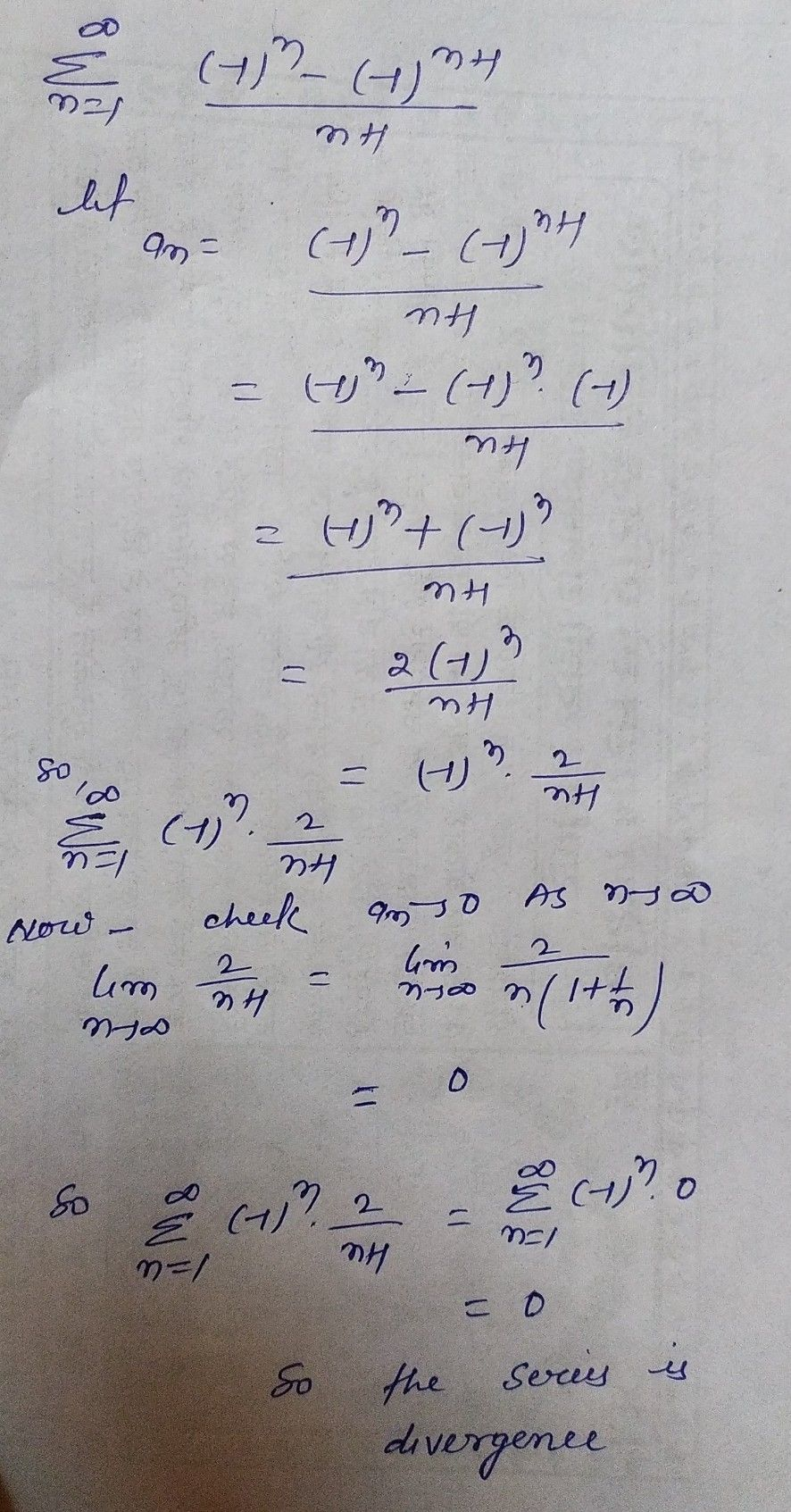Symbol
Problem$\left(b\right)$ Test the convergence of the series $:$ $8$ $\sum ^{\infty }$ $\dfrac {\left(-1\right)^{n}-\left(-1\right)^{n+1}} {n+1}$ $\left(1\right)$ $n=|$
1st-6th grade
Other
Search count: 107
SolutionQanda teacher - shahnawaz7sorry convergence inplace of divergence